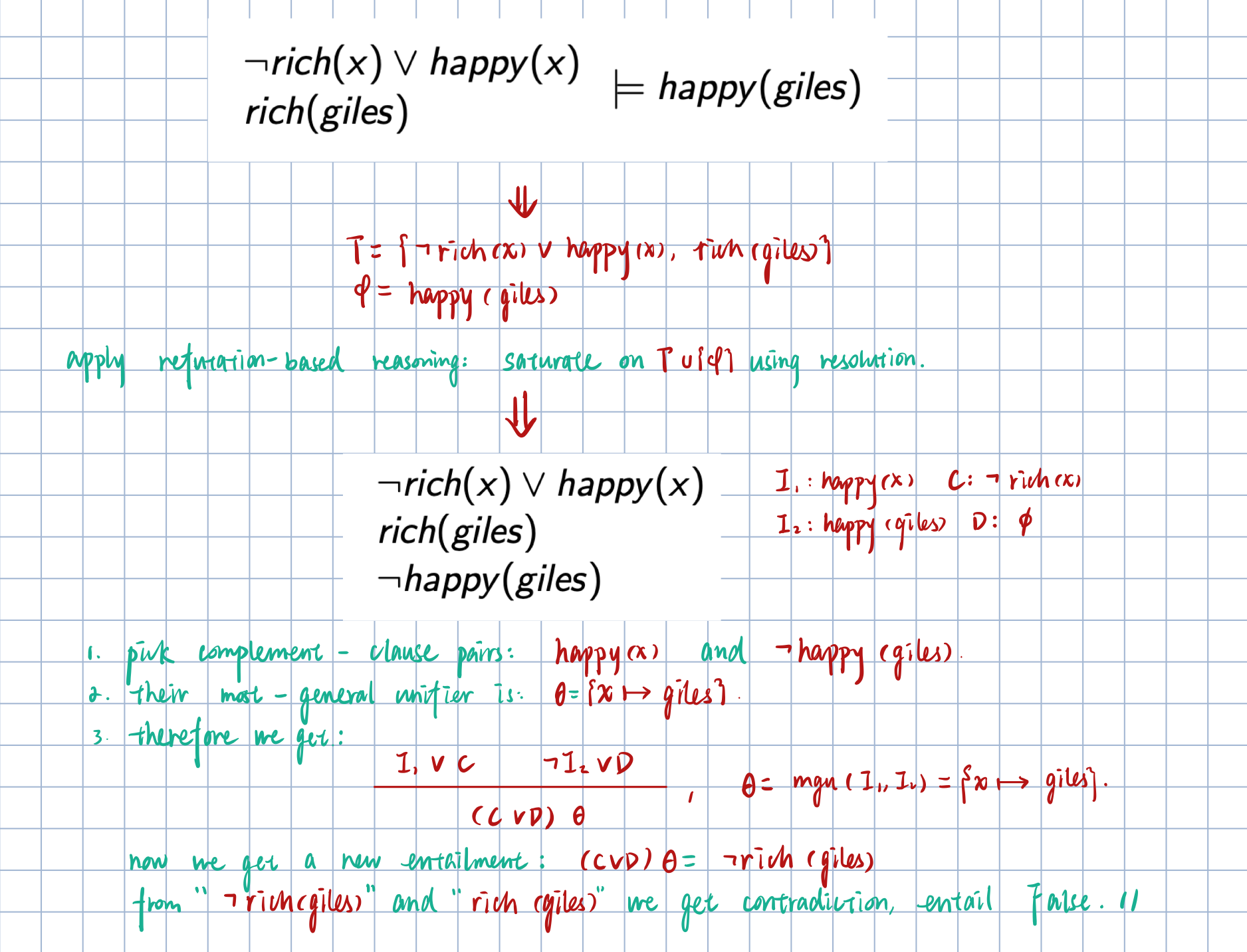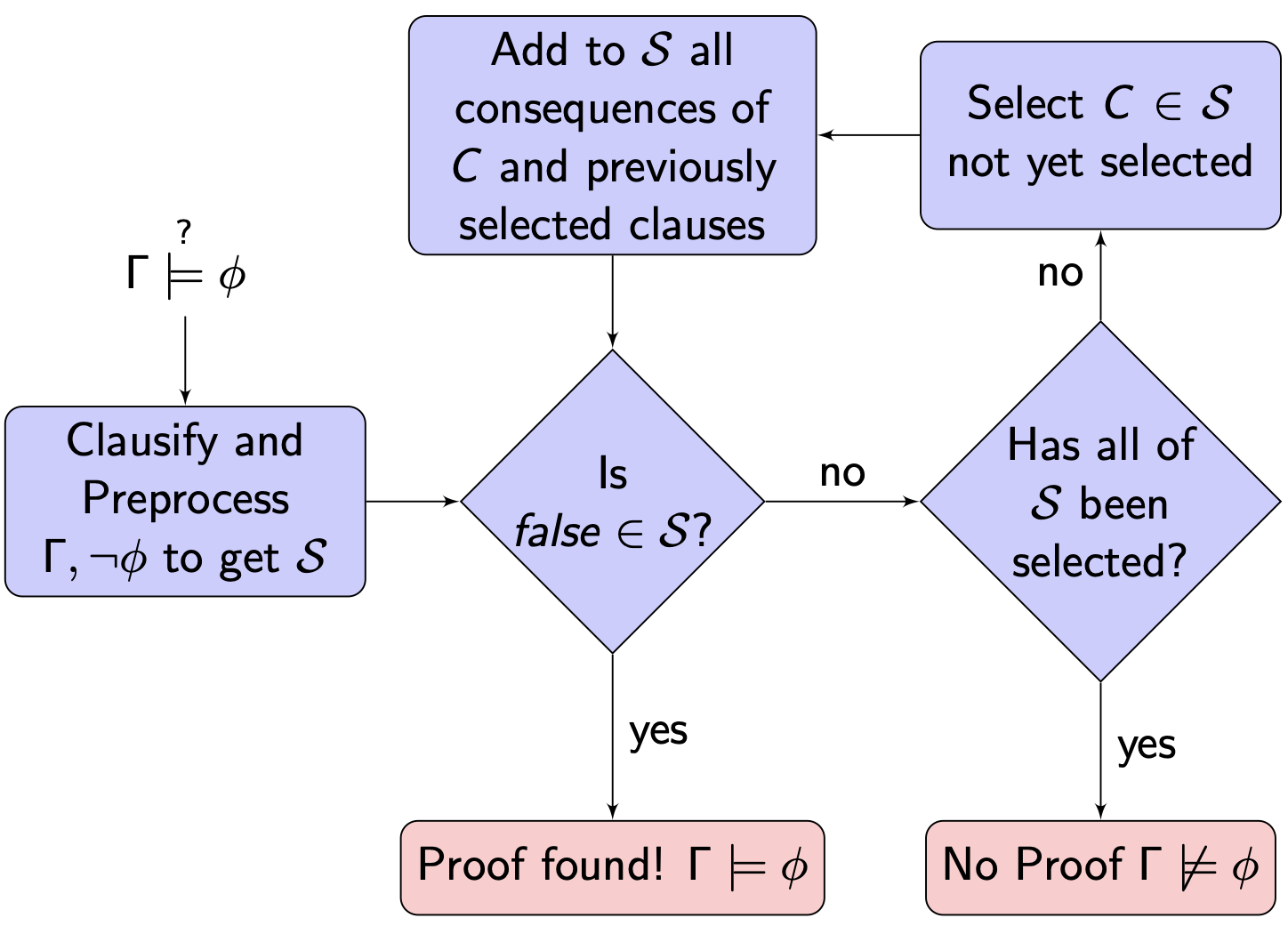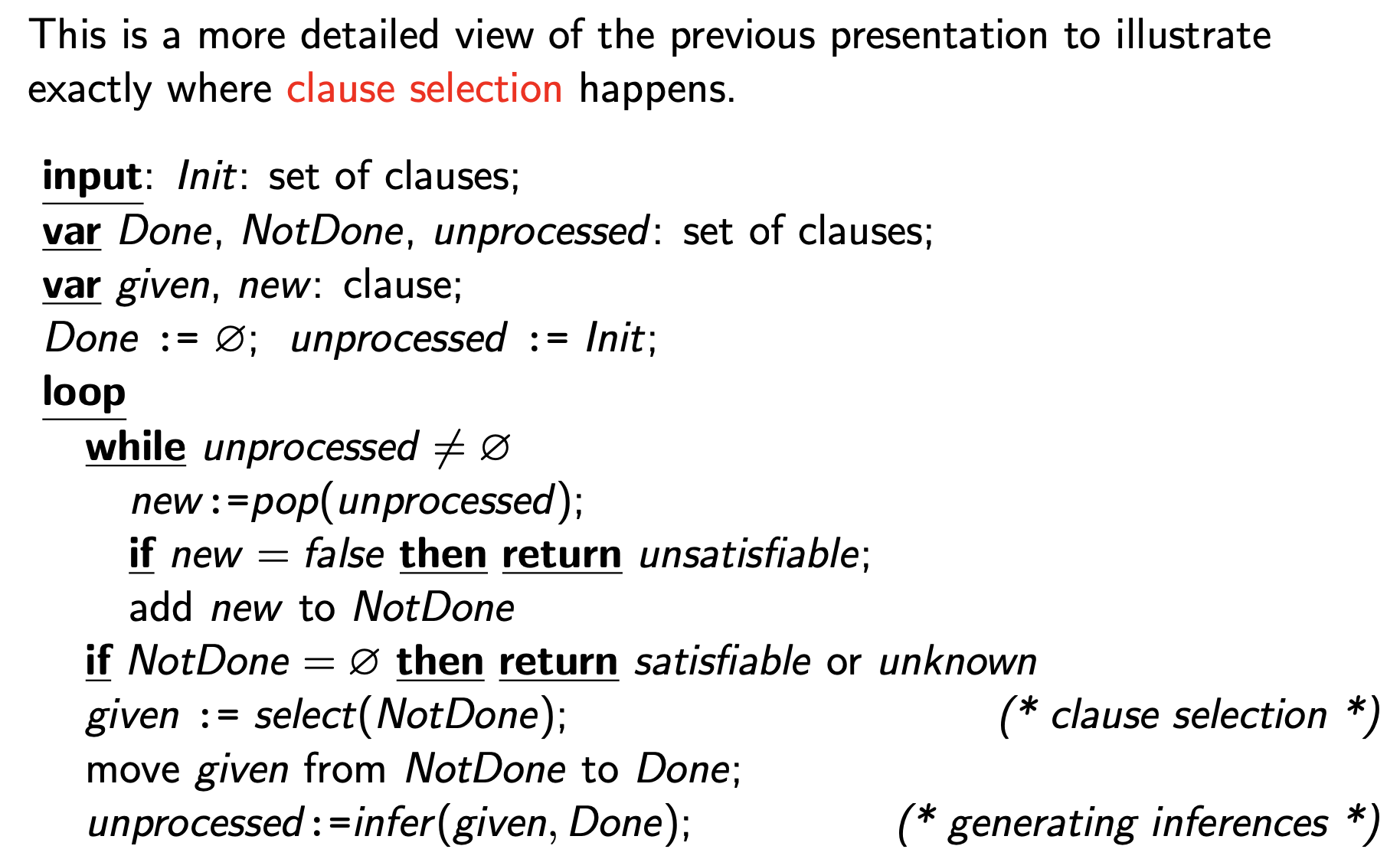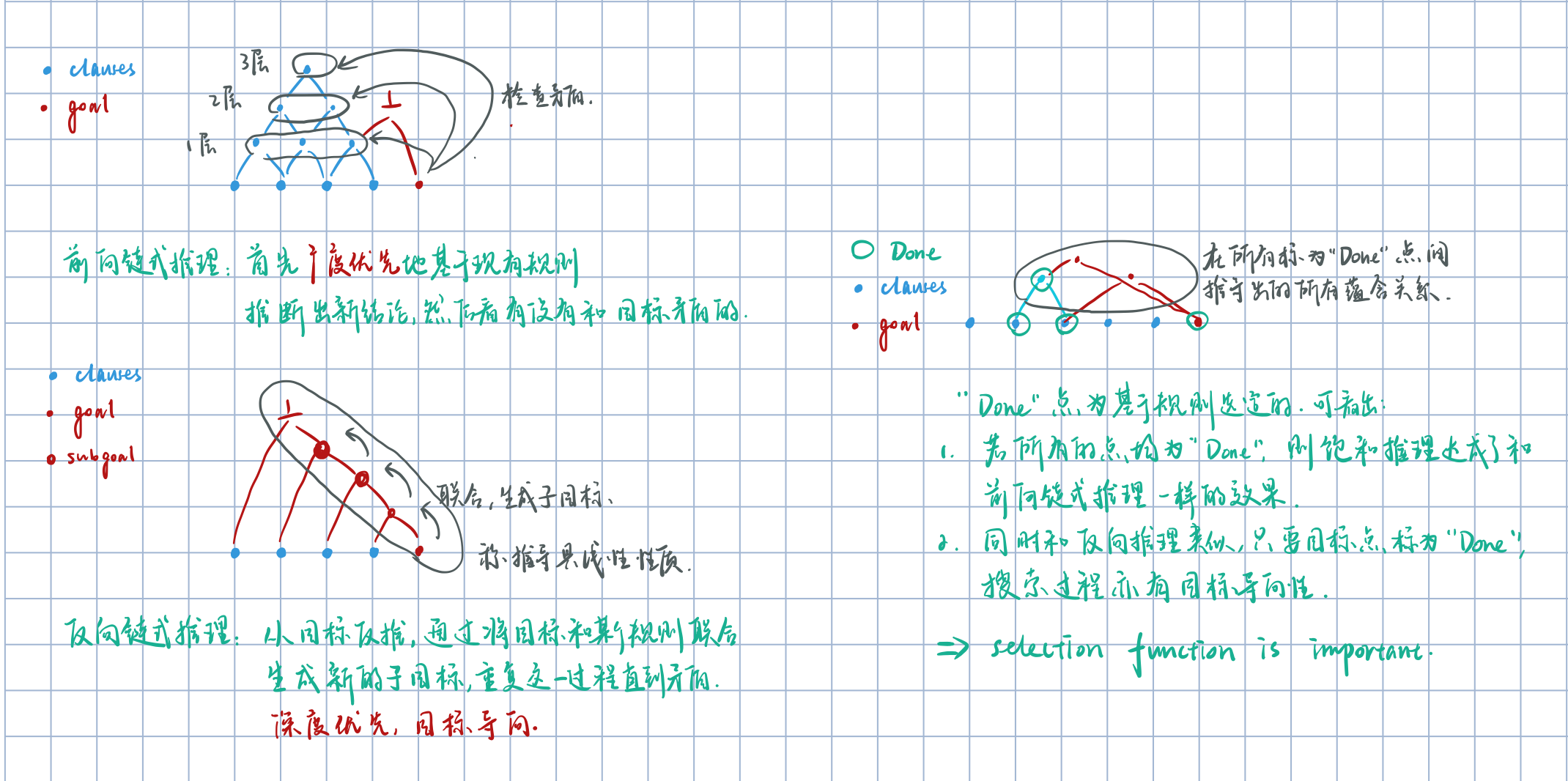# 基于知识组织的人工智能:一阶逻辑推理

## Chapter 3： 一阶逻辑推理

Posted by R1NG on February 22, 2022 Viewed Times

# 一阶逻辑推理

## 1. 一阶逻辑推理的一般框架: 饱和 (Saturation) 与 子句化 (Clausification)

### 1.1 基于反驳的推理

1. 文字 指原子或原子的取反 (Negation).
2. 子句 指一系列文字的析取, 隐式地被全称量词量化.
3. 称两个原子 $l_1$, $l_2$ 联合, 若存在替换 $\theta$ 使 $l_1 \theta, l_2 \theta$ 在语法上相同.
4. 若某两个原子 $l_1, l_2$ 能够联合, 则必存在一个 最一般的联合子 $mgu(l_1, l_2)$.
5. 归结原则 (Resolution) 可用于具备下列形式的子句上:

$\frac{\neg I_1 \vee C ~~~ I_2 \vee D}{(C \vee D)\theta}, ~~ \theta = \text{mgu}(I_1, I_2).$

且可以证明归结原则是 正确的 (sound), 因为结论对任何前件条件 (Premises) 的模型都成立.

6. 一般地, 为了避免命名冲突, 我们需要在对前件条件应用归结原则前将它们重命名以确保不存在名称相同的变量.

$\Gamma \vDash \phi$

$\Gamma \nvDash \phi.$

$\Gamma \vDash \phi.$### 1.2 饱和

$\Gamma \vDash \phi$

1. 将 $S$ 拆分为待蕴含的 NotDone 和已经过蕴含处理, 不会再得出新结论的陈述组成的集合 Done 两个部分.

在初始化时, 自然地将 NotDone 初始化为

$\Gamma \cup \{\neg \phi\}$

并将 Done 初始化为 $\emptyset$.

2. NotDone 经过转化和预处理 变为一系列子句组成的集合.
3. 检查经过转换后的集合, 若已经发现 False 则直接返回 valid.
4. 若不能直接从第三步中得出结论, 则从 NotDone基于某些规则 选取一个子句 $C$, 将其移入 Done.
5. 执行 $C$ 与 Done 中任何其他子句之间所能执行的所有的推导规则 (Inference), 如归结原则, 并将生成的所有有意义的新结论放到 NotDone 中以备后续检查.
6. 检查 NotDone 集合是否为空. 若不为空的话, 返回到第三步.
7. 最后, 如果直到所有的定理及其归纳出的衍生结论均被检查后仍未归纳出 False, 则返回 not valid.1. 饱和推理是 有目标的 (Goal-Directed): 和前向链式推理 盲目地 尽可能地推导知识库所蕴含的新结论不同, 从一开始饱和推理的目标就是 $\text{goal}(\neg \phi)$.

因此, 饱和推理在达成目标之后就会立即终止并返回结果, 并且还可以反向推理.

2. 饱和推理对搜索路径中的新节点的选择是 基于最优标准 (Best-First) 的.## 2.

REFERENCES:

Forward Logic Programming